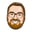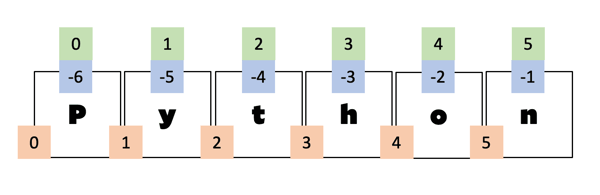Related Tags

python

# How slicing works in PythonBas Steins

### Overview

In Python, some objects like str or list can be sliced. For example, you can get the first element of a list or a string using the following code:

my_list = [1,2,3]
print(my_list) # element at index 0 will be printed

my_string = "Python"
print(my_string) # element at index 0 will be printed

Slicing in Python

Python uses square brackets—[ and ]to access single elements of objects that can be decomposed into parts.

However, there is more to the inside of these square brackets than just accessing individual elements:

### Negative indexing

Perhaps you already know that you can use negative indices in Python, as shown below:

my_list = list("Python")
print(my_list[-1]) # element at last index will be printed

my_list = list("Python")
print(my_list[-2]) # element at second last index will be printed

Negative Indexing in Python

Something like my_list[-1] represents the last element of a list, my_list[-2] represents the second last element and so on.

### The colon

What if you want to retrieve more than one element from a list? Say you want everything from start to end except for the very last one. In Python, we can do the following:

my_list = list("Python")
print(my_list[0:-1])

Slicing in Python using Colon

What if you want every even element of your list, for example, element 0, 2, and so on? For this, we need to go from the first element to the last element but skip every second item:

my_list = list("Python")
print(my_list[0:len(my_list):2]) 
Skipping indexes while slicing

### The slice object

Behind the scenes, the index we use to access individual items of a list-like object consists of three values—(start, stop, step). These objects are called slice objects and can be manually created with the built-in slice function.

We can check if the two are indeed the same:

my_list = list("Python")
start = 0
stop = len(my_list)
step = 2
slice_object = slice(start, stop, step)
print(my_list[start:stop:step] == my_list[slice_object])

Inner working of the slice functionHave a look at the illustration above. The letter P is the first element in our list, thus it can be indexed by 0 (see the numbers in the green boxes). The list has a length of 6, and therefore, the first element can alternatively be indexed by -6 (negative indexing is shown in the blue boxes).

The numbers in the green and blue boxes identify single elements of the list. The numbers in the orange boxes determine the slice indices of the list. If we use the slice’s start and stop, every element between these numbers is covered by the slice. Following are some examples:

print("Python"[0:1])
print("Python"[0:5])


That’s just an easy way to remember that the start value is inclusive and the end value is exclusive.

### Sane defaults

Most of the time, you want to slice your list by

• Starting at 0
• Stopping at the end
• Stepping with a width of 1

Therefore, these are the default values and can be omitted in our : syntax:

my_list = [1,2,5,7,9]
print(my_list[0:-1] == my_list[:-1])
print(my_list[0:len(my_list):2] == my_list[::2])


Technically, whenever we omit a number between colons, the omitted ones will have the value of None.

And in turn, the slice object will replace None with

• 0 for the start value
• len(list) for the stop value
• 1 for the step value

However, if the step value is negative, the None values are replaced with

• -1 for the start value
• -len(list) - 1 for the stop value

For example, "Python"[::-1] is technically the same as "Python"[-1:-7:-1]

### Special case: copy

There is a special case for slicing, which can be used as a shortcut sometimes.

If you use just the default values, such as my_list[:], it will give you the exact same items:

my_list = list("Python")
my_list_2 = my_list[:]
print(my_list==my_list_2)


The elements in the list are the same. However, the list object is not. We can check that by using id:

my_list = list("Python")
my_list_2 = [1,2,5,7,9]
print(id(my_list))
print(id(my_list_2))


Note that every slice operation returns a new object. A copy of our sequence is created when using just [:].

Here are two code snippets to illustrate the difference:

a = list("Python")
b = a
a[-1] = "N"
print(a)
# ['P', 'y', 't', 'h', 'o', 'N']
print(b)
# ['P', 'y', 't', 'h', 'o', 'N']

a = list("Python")
b = a[:]
a[-1] = "N"
print(a)
# ['P', 'y', 't', 'h', 'o', 'N']
print(b)
# ['P', 'y', 't', 'h', 'o', 'n']


### Examples

Some often used examples:

 Use case Python Code Every element no slice, or [:] for a copy Every second element [::2] (even) or [1::2] (odd) Every element but the first one [1:] Every element but the last one [:-1] Every element but the first and the last one [1:-1] Every element in reverse order [::-1] Every element but the first and the last one in reverse order [-2:0:-1] Every second element but the first and the last one in reverse order [-2:0:-2]

### Assignments

p = list("Python")
# ['P', 'y', 't', 'h', 'o', 'n']
p[1:-1]
# ['y', 't', 'h', 'o']
p[1:-1] = 'x'
print(p)
['P', 'x', 'n']

p = list("Python")
p[1:-1] = ['x'] * 4
print(p)
# ['P', 'x', 'x', 'x', 'x', 'n']


### Understanding the loop

Every slice object in Python has an indices method. This method returns a pair of start, end, and step with which we can rebuild a loop equivalent to the slicing operation. Sounds complicated? Let’s have a closer look.

sequence = list("Python")


Next, we create a slice object. Let’s take every second element, [::2].

my_slice = slice(None, None, 2) # equivalent to [::2].


Since we’re using None, the slice object needs to calculate the actual index values based on the length of our sequence. Therefore, to get our index triple, we need to pass the length to the indices method:

indices = my_slice.indices(len(sequence))


This will give us the triple (0, 6, 2). We now can recreate the loop:

sequence = list("Python")
start = 0
stop =  6
step =  2
i = start
while i != stop:
print(sequence[i])
i = i+step


This accesses the same elements of our list as the slice operator itself would do.

### Making own classes sliceable

Python wouldn’t be Python if you could not use the slice object in your own classes. Even better, slices do not need to be numerical values. We could build an address book which sliceable by alphabetical indices.

import string
def __init__(self):

letters = letters.upper()

def __getitem__(self, key):
if isinstance(key, str):
if isinstance(key, slice):
start, stop, step = key.start, key.stop, key.step
letters = (string.ascii_uppercase[string.ascii_uppercase.index(start):string.ascii_uppercase.index(stop)+1:step])

print(string.ascii_uppercase)
print(string.ascii_uppercase.index("A"))
print(string.ascii_uppercase.index("Z"))



#### The get_addresses_by_first_letters method

    def get_addresses_by_first_letters(self, letters):
letters = letters.upper()


This method filters all addresses belonging to a name starting with any letter in the letters argument. First, we make the function case insensitive by converting our letters to uppercase. Then, we use a list comprehension over our internal addresses list. The condition inside the list comprehension tests if any of the provided letters matches the first letter of the corresponding name value.

#### The __getitem__ method

To make our AddressBook objects sliceable, we need to overwrite Python’s magic double underscore method, __getitem__.

    def __getitem__(self, key):
if isinstance(key, str):
if isinstance(key, slice):
start, stop, step = key.start, key.stop, key.step
letters = (string.ascii_uppercase[string.ascii_uppercase.index(start):string.ascii_uppercase.index(stop)+1:step])


At first, we check if our key is a str. This will be the case if we access our object with a single letter in square brackets like address_book["A"]. We can just return any addresses whose name starts with the given letter for this trivial case.

The interesting part is when the key is a slice object. For example, access like address_book["A":"H"] would match that condition. First, we identify all letters alphabetically between A and H. The string module in Python lists all letters in in string.ascii_uppercase. We use a slice to extract the letters between the given letters. Note that +1 in the second slice parameter. This way, we ensure that the last letter is inclusive, not exclusive.

After we determined all letters in our sequence, we use get_addresses_by_first_letters, which we already discussed. This gives us the result we want.

RELATED TAGS

python

CONTRIBUTORBas Steins
RELATED COURSES

View all Courses

Keep Exploring

Learn in-demand tech skills in half the time